Home / Blog / Data Science Digital Book / Graphical Representations

# Graphical Representations

• July 15, 2023
• 2283
• 22### Meet the Author : Mr. Bharani Kumar

Bharani Kumar Depuru is a well known IT personality from Hyderabad. He is the Founder and Director of Innodatatics Pvt Ltd and 360DigiTMG. Bharani Kumar is an IIT and ISB alumni with more than 17 years of experience, he held prominent positions in the IT elites like HSBC, ITC Infotech, Infosys, and Deloitte. He is a prevalent IT consultant specializing in Industrial Revolution 4.0 implementation, Data Analytics practice setup, Artificial Intelligence, Big Data Analytics, Industrial IoT, Business Intelligence and Business Management. Bharani Kumar is also the chief trainer at 360DigiTMG with more than Ten years of experience and has been making the IT transition journey easy for his students. 360DigiTMG is at the forefront of delivering quality education, thereby bridging the gap between academia and industry.

### Univariate Analysis - Analysis of a single variable is called Univariate Analysis.

Graphs using which we can visualize single variables are:

• Bar Plot
• Index Plot
• Dot Plot
• Strip Plot
• Violin Plot
• Stem & Leaf Plot
• Candle Plot
• Pie Chart
• Time Series Plots
• Histogram
• Density Plot
• Boxplot or Box & Whisker Plot
• Q-Q Plot or
• Quantile - Quantile Plot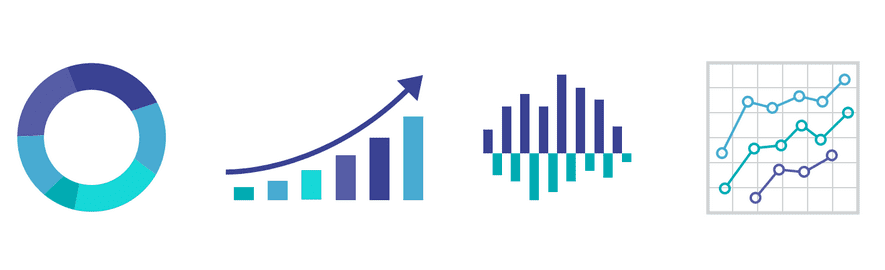## Graphical Representations

For univariate analysis, the histogram, box plot, and Q-Q plot are the most often used plots.

## Histogram

Another name for a histogram is a Frequency Distribution Plot.

The histogram's main use is to show the distribution's shape.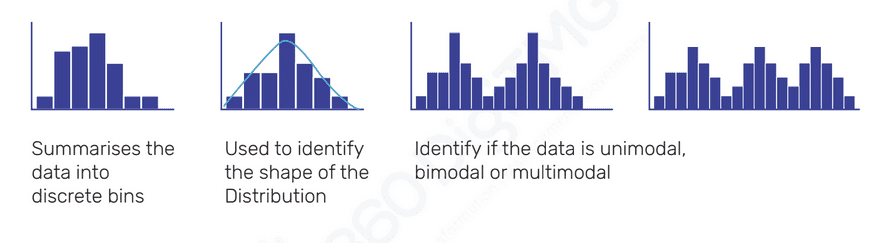Histograms are used to detect the existence of outliers as a secondary purpose.## Box Plot is also called as Box and Whisker Plot

• Box Plot gives the 5 point summary, namely, Min, Max, Q1 / First Quartile, Q3 / Third Quartile, Median / Q2 / Second Quartile
• Middle 50% of data is located in the Inter Quartile Range (IQR) = Q3 - Q1
• Formula used to identify outliers is Q1 - 1.5 (IQR) on the lower side and Q3 + 1.5 (IQR) on the upper side
• Primary Purpose of Boxplot is to identify the existence of outliers
• Secondary Purpose of Boxplot is to identify the shape of distribution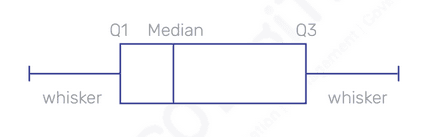## Q-Q plot is also called Quantile Quantile Plot

• Q-Q plot is used to check whether the data are normally distributed or not. If data are non-normal then we resort to transformation techniques to make the data normal
• The line in the Q-Q plot connects from Q1 to Q3
• X-axis contains the standardized values of the random variable
• Y-axis contains random values, which are not standardized
• If the data points fall along the line then data are considered to be Normally Distributed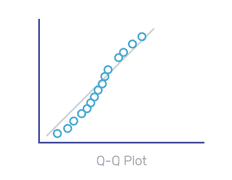## Bivariate Analysis

Analysing two variables is known as bivariate analysis.

To determine whether two variables are correlated, use a scatter plot.

The primary purpose of the Scatter Plot is to determine the following:

• Direction - Whether the direction is Positive or Negative or No Correlation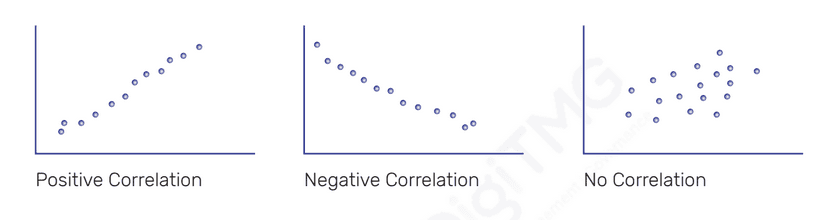• Strength - Whether the strength is Strong or Moderate or Weak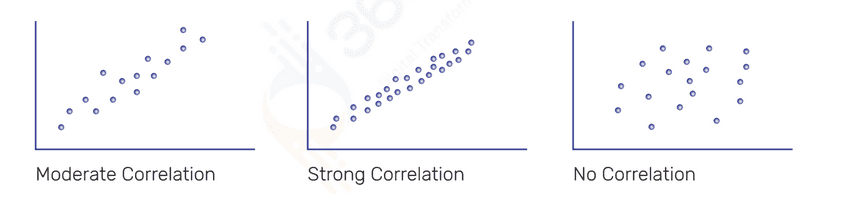• Check whether the relationship is Linear or Nonlinear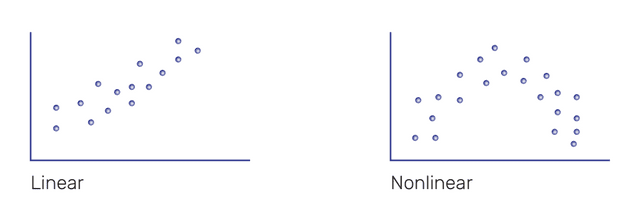Finding out if the relationship is linear or non-linear is the Scatter Plot's secondary goal.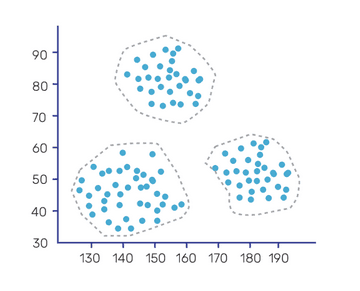• Determining strength using a scatter plot is subjective
• Objectively evaluate strength using Correlation Coefficient (r)
• Correlation coefficient value ranges from +1 to -1
• Covariance is also used to track the correlation between 2 variables
• However, Correlation Coefficient normalizes the data in correlation calculations whereas Covariance does not normalize the data in correlation calculation
• |r| > 0.85 implies that there is a strong correlation between the variables
• |r| < = 0.4 implies that there is a weak correlation
• |r| > 0.4 & |r|< = 0.85 implies that there is a moderate correlation

## Multivariate Analysis

The two main plots to perform Multivariate analysis are:

• Pair Plot
• Interaction Plot

Click here to learn Data Science Course, Data Science Course in Hyderabad, Data Science Course in Bangalore

### Data Science Training Institutes in Other Locations